# Isolability analysis and sensor placement

09 Mar 2017

This tutorial describes how to perform basic isolability analysis for a model and sensor placement analysis.

Again, as in a previous tutorial an idealized model of an electric motor will be used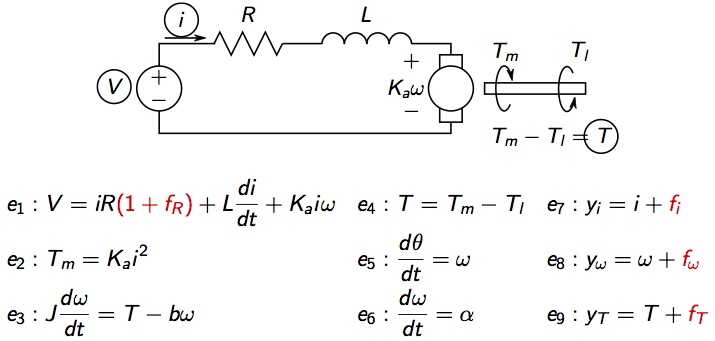where V is voltage, i current, Tm motor torque, Tl load torque, omega rotational speed, theta motor angle. There are four considered faults, three sensor faults and a change in the internal resistance.

Follow the previous tutorial to define the model. After the model has been defined, use the class method `Lint` to show basic model information

and the model structure can be shown using the `PlotModel` class method

which produces the figure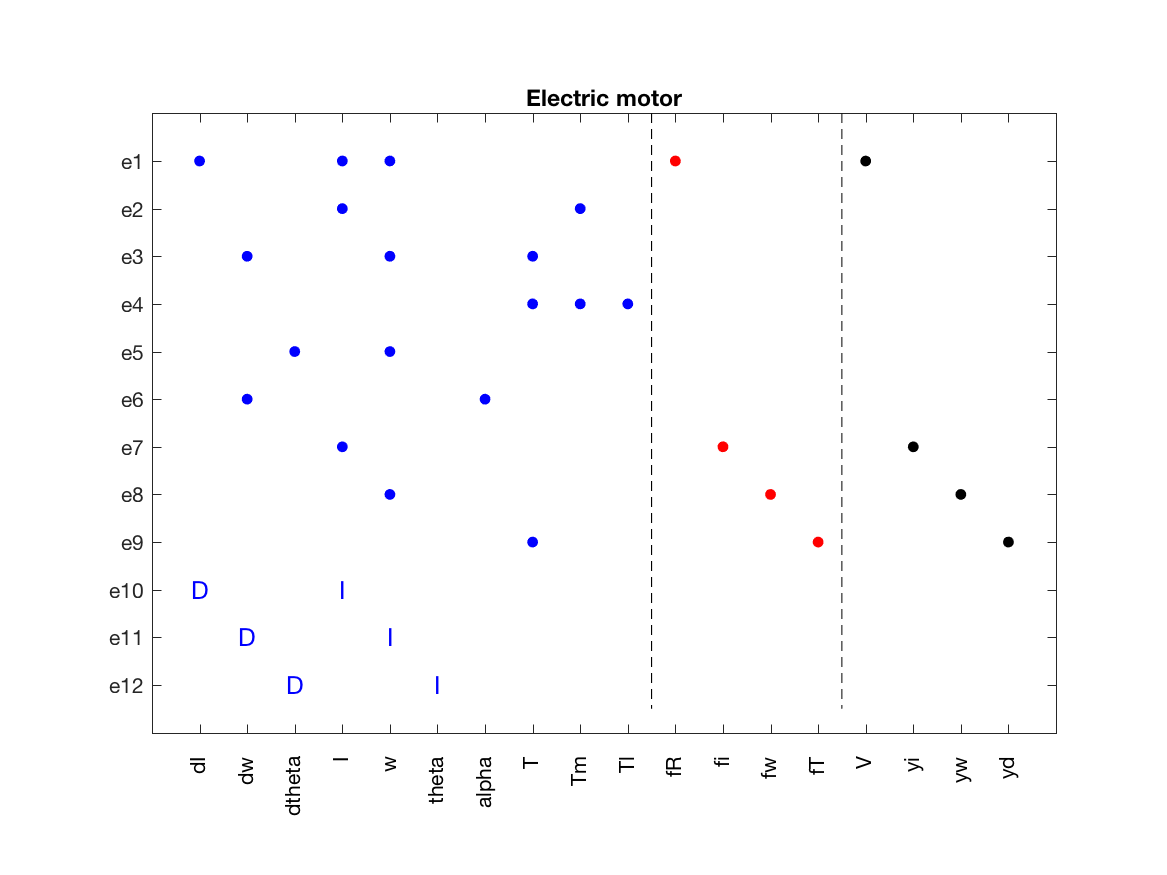## Isolability analysis

The model is over-constrained, i.e., more equations than unknown due to the three measurement equations already included. This means that there are redundancy in the model and there is a possibility to create residuals for fault detection and fault isolation. Before designing residuals, it is possible to analyze the model and see what diagnosis performance that is ideally possible with the used sensors. This is called _isolability analysis``` and can be performed using the class method ```IsolabilityAnalysis` as

which produces the figure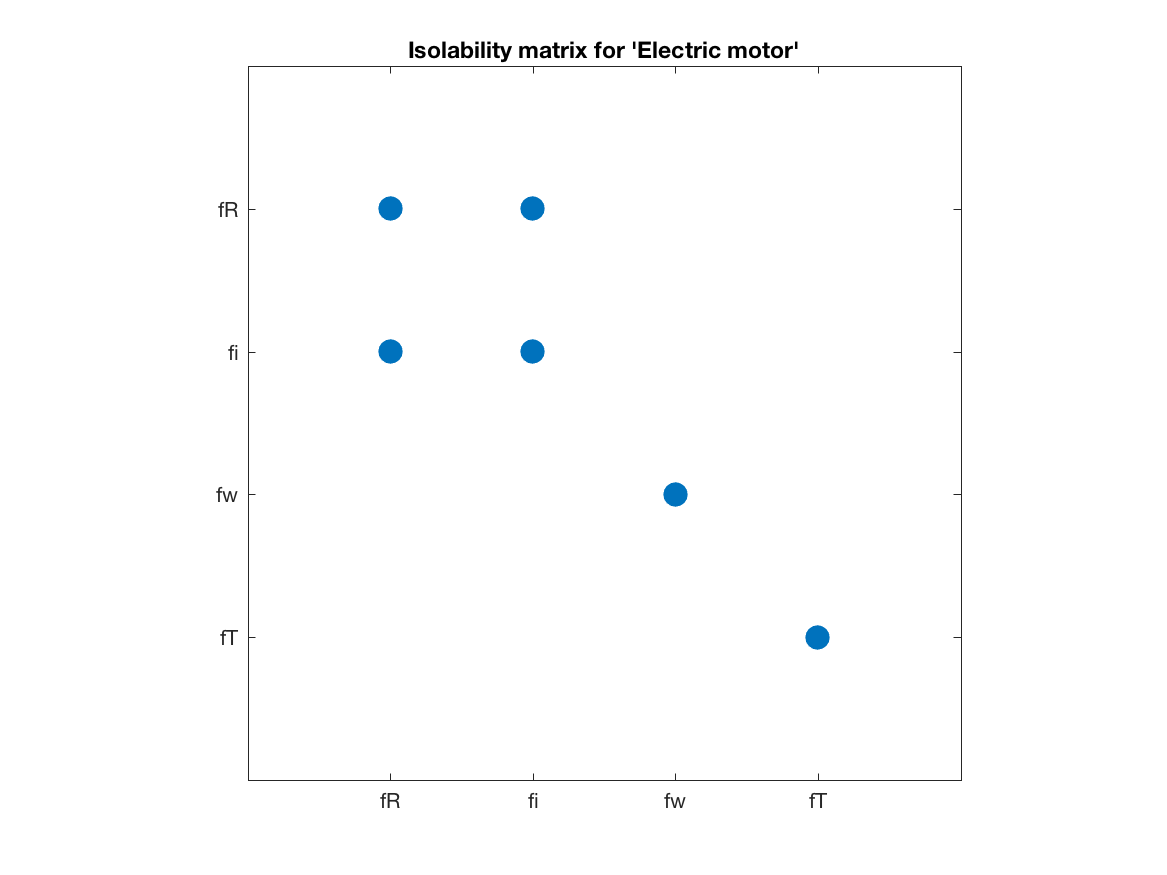This figure illustrates the isolability matrix, where a dot in position (i,j) indicates that, structurally, fault fi can not be isolated from fault fj. The ideal performance is this a diagonal matrix. Here it is clear that all faults can be detected, faults fw and fT can be uniquely isolated, and faults fR and fi can be isolated from the other two but can not be isolated from each other.

## Sensor placement for full isolability

Assume we are considering adding a sensor to be able to achieve ideal isolability performance, a sensor placement analysis tells us which are possible sensor positions. First, tell the model which are the possible sensor locations, and if any new sensors may become faulty. First, consider the case where all unknowns are possible sensor locations and non may become faulty. This done in Matlab using the class methods `PossibleSensorLocations` and `SensorLocationsWithFaults` as

Now, perform the sensor placement analysis using the class method `SensorPlacementIsolability` as

The variable `sens` now include all minimal sensor sets that achieves full isolability (if possible). In this case, there are three minimal solutions

Let’s add the current sensor, and do another isolability analysis to see that we have actually obtained ideal performance

which produces the isolability matrix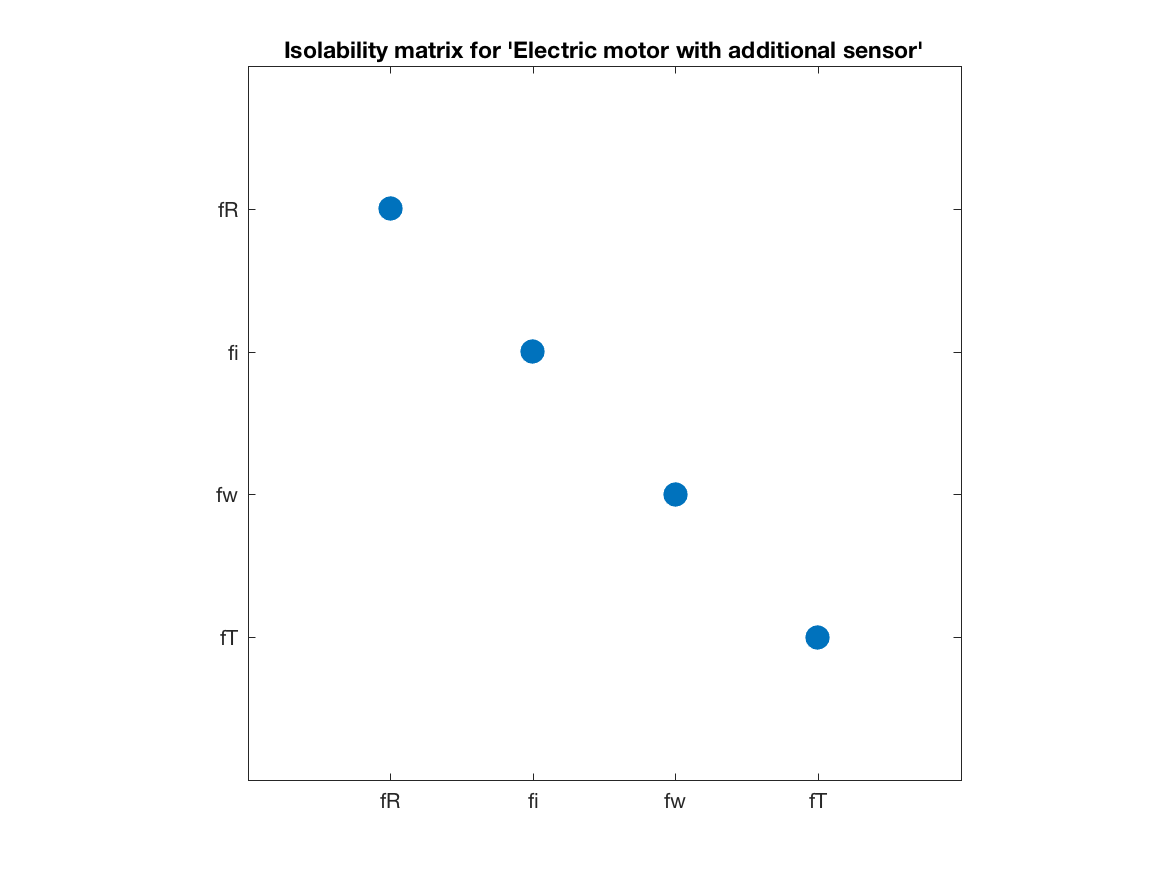## Sensor placement where new sensors may fail

Now, assume that also the new sensors may fail. Tell that to the model and redo the sensor placement analysis

It turns out that you get the same set of solutions, in this case only this is not a general property. To verify the solution, add the sensor and redo the sensor placement analysis by

which produces the isolability matrix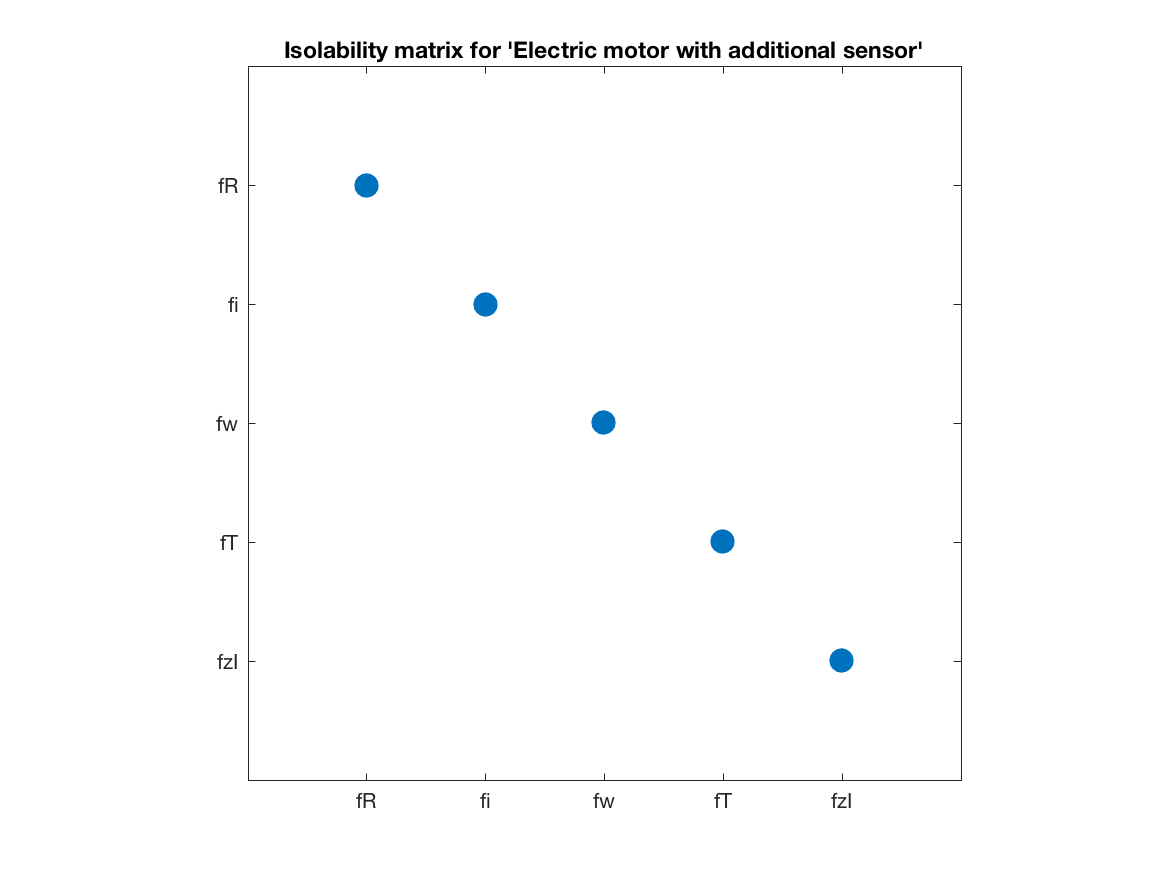which is ideal and note that a new fault has appeard in the analysis, this is the fault in the new sensor.

Categories:

Updated: